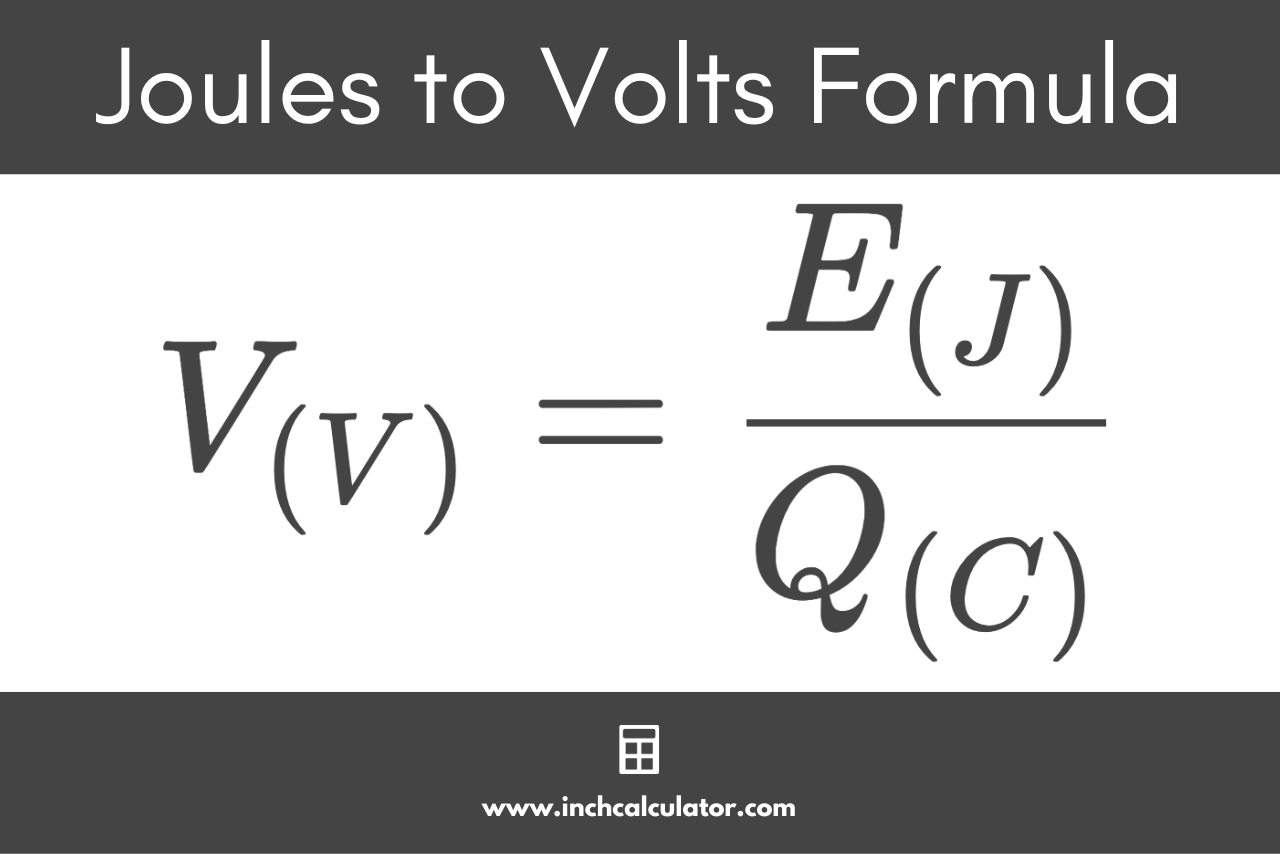# Joules to Volts Conversion Calculator

Convert joules to volts by entering the energy in joules and the charge in coulombs below.

J
C

## Volts Results:

V
Learn how we calculated this below

## How to Convert Joules to Volts

Joules are a measure of electrical energy dissipated to move a 1-kilogram object one meter using a constant force of one newton. Coulombs are the amount of charge accumulated in one second by a current of one ampere.

Voltage is the measure of the electric potential in an electric circuit to move an electric charge and is measured in volts.

You can convert joules to volts using these measurements using a formula.

### Joules to Volts Formula

To convert energy in joules to voltage in volts, use the formula below.

V(V) = E(J) / Q(C)

Thus, the voltage V is equal to the energy E in joules divided by the charge Q in coulombs.For example, let’s convert 20 joules of energy with a charge of 5 coulombs to volts.

V(V) = 20 J / 5 C
V(V) = 4 V

You might also be interested in our joules to watts calculator.